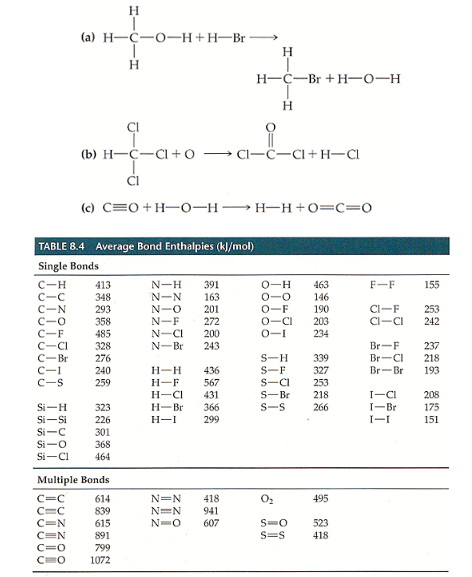# Problem: Using bond enthalpies (Table 8.4), estimate ΔH for the following gas-phase reactions.

###### FREE Expert Solution
98% (354 ratings)
###### FREE Expert Solution

Calculation of ΔH using bond energies or bond enthalpies:

ΔHrxn = reactants - products

98% (354 ratings)###### Problem Details

Using bond enthalpies (Table 8.4), estimate ΔH for the following gas-phase reactions.Frequently Asked Questions

What scientific concept do you need to know in order to solve this problem?

Our tutors have indicated that to solve this problem you will need to apply the Bond Energy concept. You can view video lessons to learn Bond Energy. Or if you need more Bond Energy practice, you can also practice Bond Energy practice problems.

What is the difficulty of this problem?

Our tutors rated the difficulty ofUsing bond enthalpies (Table 8.4), estimate ΔH for the follo...as medium difficulty.

How long does this problem take to solve?

Our expert Chemistry tutor, Dasha took 8 minutes and 13 seconds to solve this problem. You can follow their steps in the video explanation above.

What professor is this problem relevant for?

Based on our data, we think this problem is relevant for Professor Caldwell's class at UCONN.